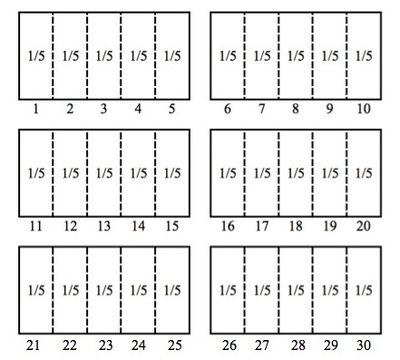# Origami Stars

Alignments to Content Standards: 5.NF.B.7.b

Avery and Megan are cutting paper to make origami stars. They need $\frac{1}{5}$ of a sheet of paper in order to make each star. If they have 6 sheets of paper, how many stars can they make? Explain your work and draw a picture to support your reasoning.

## IM Commentary

The purpose of this task is to present students with a situation in which they need to divide a whole number by a unit fraction in order to find a solution. Calculating the number of origami stars that Avery and Megan can make illustrates students' understanding of the process of dividing a whole number by a unit fraction.

This task is more concrete that some of the other division problems: note that the picture drawn reflects the situation very literally: the rectangles in the picture represent rectangular pieces of paper. Compare this with the solutions for 5.NF Banana Pudding where the rectangles in the picture represent cups or 5.NF Dividing by $\frac12$ where the rectangles in the picture represent sandwiches, quarts of soup, or pounds of gold.

## Solution

In order to find out how many origami stars Avery and Megan can make, we need to find out how many pieces equivalent to $\frac{1}{5}$ of a sheet of paper we can divide the 6 pieces of paper into. This means that the answer to this question will be the solution to the following division problem: $$6\div\frac{1}{5}=?$$ Based on our understanding of fractions, we know that there are 5 pieces of that are equivalent to $\frac{1}{5}$ of a sheet in each sheet of paper. This means that there are $6\times5=30$ pieces of paper with size $\frac{1}{5}$ of a sheet in the 6 sheets of paper that Avery and Megan have to make their stars. We know that this is true because $30\times\frac{1}{5}=6$. Based on our understanding of the relationship between multiplication and division, this tells us that $6\div\frac{1}{5}=30$. Thus, Avery and Megan can make 30 origami stars. The picture below shows that this is correct:The picture above shows that there are 30 pieces of paper with size $\frac{1}{5}$ of a sheet in the 6 sheets of paper that Avery and Megan have to make their stars. This supports our reasoning and shows that Avery and Megan can make 30 origami stars.